# Problems of the surface area of a cube

#### Number of problems found: 103

• Concrete pedestalThe carpenters made wooden mold on a concrete pedestal in the shape of a cube with an edge 2 meters long. What is the area in which the concrete touches wooden molds? (No lid or bottom)
• Center of the cubeThe Center of the cube has a distance 16 cm from each vertex. Calculate the volume V and surface area S of the cube.
• Surface and volume - cubeFind the surface and volume of a cube whose wall diagonal is 5 cm long.
• Surface area of cylinder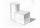Determine the lateral surface of the rotary cylinder which is circumscribed cube with edge length 5 cm.
• Cube wallsFind the volume and surface area of the cube if the area of one wall is 40cm2.
• The funnelThe funnel has the shape of an equilateral cone. Calculate the content of the area wetted with water if you pour 3 liters of water into the funnel.
• Cube and sphereCube with the surface area 150 cm2 is described sphere. What is sphere surface?
• Sphere vs cubeHow many % of the surface of a sphere of radius 12 cm is the surface of a cube inscribed in this sphere?
• Wall diagonalCalculate the length of wall diagonal of the cube whose surface is 384 cm square.
• CubeCalculate the surface of the cube ABCDA'B'C'D' if the area of rectangle ACC'A' = 344 mm2.
• Rectangle poolFind dimensions of an open pool with a square bottom with a capacity of 32 m3 to have painted/bricked walls with the least amount of material.
• Cube 5The content area of one cube wall is 32 square centimeters. Determine the length of its edges, its surface and volume.
• Body diagonalCalculate the volume and surface of the cube if the diagonal body measures ten dm.
• CalculateCalculate the surface of the cube if its volume is 64 cm ^ 3.
• Body diagonalFind the cube surface if its body diagonal has a size of 6 cm.
• The cube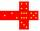The surface of the cube is 150 square centimeters. Calculate: a- the content of its walls b - the length of its edges c - its volume
• The body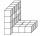The body on the figure consists of cubes with an edge length 10 cm. What surface has this body?
• Sum of the edgesThe sum of the lengths of all edges of the cube is 72 cm. How many cm2 of colored paper are we going to use for sticking?
• Funnel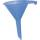The funnel has the shape of an equilateral cone. Calculate the surface wetted with water if we poured into the funnel 7.1 liters of water.
• Grid/network of cube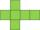The grid of the cube has a circumference of 42 mm. Calculate the surface area of the cube . ..

Do you have an exciting math question or word problem that you can't solve? Ask a question or post a math problem, and we can try to solve it.

We will send a solution to your e-mail address. Solved examples are also published here. Please enter the e-mail correctly and check whether you don't have a full mailbox.

Cube Problems. Examples for the calculation of the surface area of ​​the solid object .